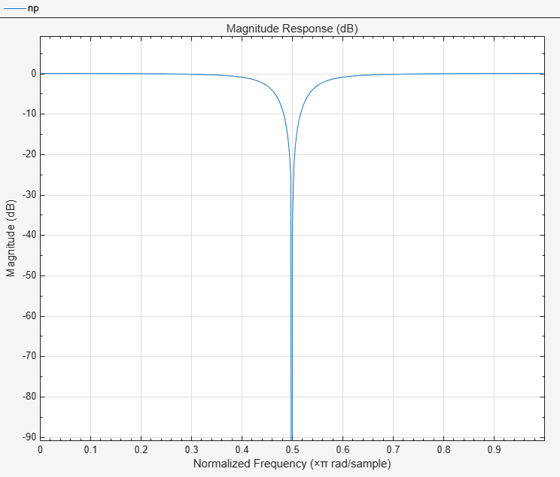getBandwidth

Get 3 dB bandwidth

Description

example

BW = getBandwidth(npFilter) returns the 3 dB bandwidth for the notch peak filter. If the Specification property is set to 'Quality factor and center frequency', the 3 dB bandwidth is determined from the quality factor value. If the Specification property is set to 'Coefficients', the 3 dB bandwidth is determined from the BandwidthCoefficient value and the sample rate.

Examples

collapse all

Create a dsp.NotchPeakFilter object with the Specification property set to 'Quality factor and center frequency'. The default quality factor Q is 5, and the center frequency Fc is 11,025 Hz.

np = dsp.NotchPeakFilter('Specification','Quality factor and center frequency')
np =
dsp.NotchPeakFilter with properties:

Specification: 'Quality factor and center frequency'
QualityFactor: 5
CenterFrequency: 11025
SampleRate: 44100

Compute the 3 dB bandwidth of the notch peak filter using the getBandwidth function. The bandwidth is computed as the ratio of the center frequency and the quality factor, $\frac{\mathrm{Fc}}{\mathit{Q}}$.

getBandwidth(np)
ans = 2205

Visualize the filter response using fvtool.

fvtool(np)Input Arguments

collapse all

Notch peak filter whose 3 dB bandwidth is measured, specified as a dsp.NotchPeakFilter object.

Output Arguments

collapse all

3 dB bandwidth of the filter, returned as a scalar.

Data Types: double

Objects

Introduced in R2014a

DSP System Toolbox Documentation평가판 신청